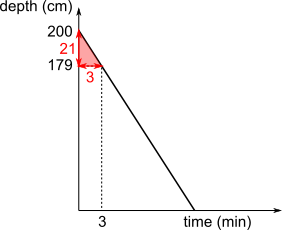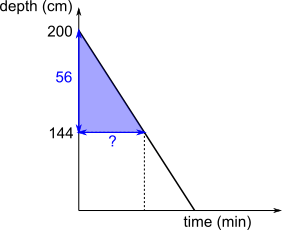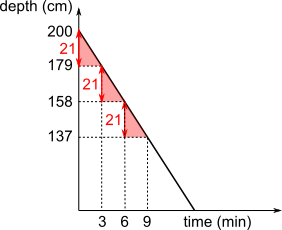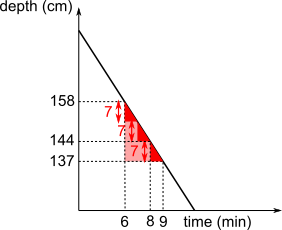#### You may also like### Consecutive Numbers

An investigation involving adding and subtracting sets of consecutive numbers. Lots to find out, lots to explore.### Calendar Capers

Choose any three by three square of dates on a calendar page...### Days and Dates

Investigate how you can work out what day of the week your birthday will be on next year, and the year after...

# Draining a Pool

##### Age 11 to 14 ShortChallenge Level

Using similar trianglesTriangles are similar so 21 : 3 = 56 : ?
21 : 3 = 7 : 1
56 = 7$\times$8 so 56 : 8 is also the same as 7 : 1, ? = 8.

Using rates of change
In the first 3 minutes, the depth of the water decreases by 21 cm.
So in each 1 minute, depth decreases by 7 cm.

To reach 144 cm, it must decrease by 35 cm more, since 144 + 35 = 179.

35 = 7$\times$5, so it will take 5 more minutes, or 8 minutes in total.In $3$ minutes, the depth of the water goes down by $21$ cm.

So the gradient of the line is $\frac{-21}3=-7$.

When the water level is $144$ cm, the depth of the water will have gone down by $56$ cm.

So, if this happens at time $t$, $\frac{-56}t=-7$, so $t=8$.

Using proportion
time decrease final depth
3 minutes 21 cm 179 cm
3 more min 21 cm 158 cm
1 more min 7 cm 151 cm
1 more min 7 cm 144 cm

Total 8 minutes.

Using congruent triangles
Because the graph is a straight line, we can use congruent triangles congruent to find other points on the graph, as shown on the right.These three triangles have taken us too far, so we can now use smaller similar triangles to find more information:We can see that after 8 minutes, the height of the water will be 144 cm.

You can find more short problems, arranged by curriculum topic, in our short problems collection.